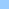# animate_gravity

how to calculate acceleration
gravityTime based animation and gravity ```From: Darrel Plant Subject: Re: Time based animation and gravity - Help! To: DIRECT-L@LISTSERV.UARK.EDU At 4:07 PM -0700 8/10/00, Martin Pallett wrote: 1. How do you calculate acceleration in a time based animation? the basic formula I am using for the linear animation is newloc = elapsedTime * vector / overallTime + startloc -- thanks to Darrel Plant but I am stumped when it comes to including the math involved in the acceleration Gravity acceleration is itself a time-based formula, the standard simple form is: accel (t) = 32 ft / (t * t) (or 9.8m / (t * t) ) That's usually referred to as g. Acceleration doesn't tell the whole story, though. What's more useful is the distance travelled in free fall: distance (t) = g / 2 * (t * t) or in feet: distance (t) = 16 (t * t) Pretty simple. You'll probably want to futz around with the value you multiply t squared by, unless you've got other animations conforming to real units, this is probably not exactly what you want. 2. Should overallTime include the time taken for any bounces? or should it be tacked on afterwards as a result of velocity at impact? Unless you're making a simulator, just do what looks best. There's lots of pages on the physics of free-falling bodies. Here's one: http://www.mathcs.emory.edu/ccs/math190g/ModuleII/ModIIP5.html ```Home shock + cgi Bits 'n pieces Director Lingo ShockLets Contact